# Electronic Devices - Op-Amp Applications

Exercise : Op-Amp Applications - General Questions
6.
Calculate the output voltage if V1 = V2 = 0.15 V.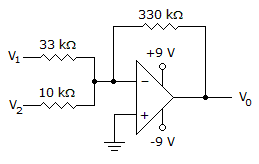0 V
4.65 V
6.45 V
–6.45 V
Explanation:
No answer description is available. Let's discuss.

7.
Determine the output voltage for this circuit with a sinusoidal input of 2.5 mV.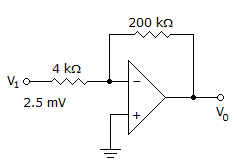–0.25 V
–0.125 V
0.25 V
0.125 V
Explanation:
No answer description is available. Let's discuss.

8.
Determine the output voltage when V1 = V2 = 1 V.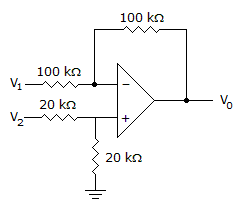0 V
–2 V
1 V
2 V
Explanation:
No answer description is available. Let's discuss.

9.
How many op-amps are required to implement this equation?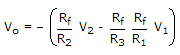2
3
4
1
Explanation:
No answer description is available. Let's discuss.

10.
Calculate the input voltage if the final output is 10.08 V.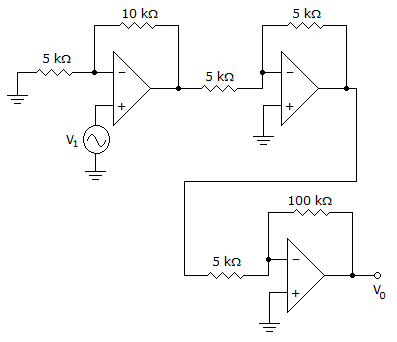–1.05 V
0.525 V
0.168 V
4.2 V